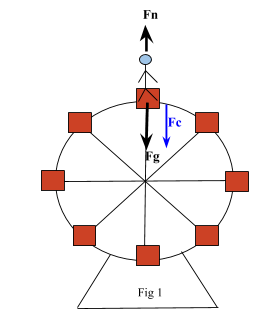# Motion in a Vertical Circle

## Summary

• Centripetal force can be defined through a formula$F\quad =\quad \frac { m{ v }^{ 2 } }{ r }$.
• Whenever an object is rotating in a vertical circle, the gravitational and centripetal forces act along the same axis at the top and bottom of the arc.
• Wheel of a vehicle and a Ferris wheel are both examples of motion in a vertical circle.
• At the top of the circular arc${ F }_{ n }\quad =\quad { F }_{ c }\quad +\quad { F }_{ g }$
• At the bottom of the arc${ F }_{ n }\quad =\quad { F }_{ c }\quad -\quad { F }_{ g }$

To recall from the article ”Motion in a Circle”, that for an object moving in a circle, there is an inward force acting upon it in order to cause its inward acceleration. This is known as Centripetal Force.

Centripetal force can be defined through a formula$F\quad =\quad \frac { m{ v }^{ 2 } }{ r }$.

We also discussed motion in a horizontal circle, whose example was the conical pendulum. Here we neglected the gravitational force because it always acted in a direction perpendicular to the centripetal force.

In other words, because the centripetal and gravitational forces are perpendicular to one another, they did not affect each other. For the Conical Pendulum:

• The vertical component is$Tcos\theta \quad =\quad mg$
• The horizontal component is$Tsin\theta \quad =\quad m{ \omega }^{ 2 }r$

Now let’s continue our discussion on uniform circular motion and this time our focus will be on Motion in a vertical circle. In such a case, things get a bit complicated.

Whenever an object is rotating in a vertical circle, the gravitational and centripetal forces act along the same axis at the top and bottom of the arc. Some real life examples of motion in a vertical circle are: the wheel of a vehicle and also a Ferris wheel.

Furthermore, let’s suppose a person is on the Ferris wheel. The ferris wheel begins to rotate at a constant speed. At the top of the circular arc, the person feels a gravitational force${ F }_{ g }$ that acts in the same direction as the centripetal force${ F }_{ c }$ (as the centripetal force always acts inwards to the circle), while the normal force${ F }_{ n }$ acts in the opposite direction.

We can work this out using the equation:${ F }_{ n }\quad =\quad { F }_{ c }\quad +\quad { F }_{ g }$

This is shown in Fig 1.On the other hand, at the bottom of the arc the person feels a gravitational force${ F }_{ g }$ that points in the opposite direction as the centripetal force${ F }_{ c }$ while the normal force${ F }_{ n }$ points in the same direction as the centripetal force. This means that the normal force is larger at the bottom than at the top.

We can work this out using the equation:${ F }_{ n }\quad =\quad { F }_{ c }\quad -\quad { F }_{ g }$

This is shown in Fig 2.#### Example #1

Q. A roller coaster is going through a loop that has a radius of 4.80 m. The roller coaster cars have a speed of 13.8 m/s at the top of the loop. During testing and development of the roller coaster, it was determined that the cars and passengers have a combined mass of 4800 kg on an average run. Determine the amount of force the track must be designed to withstand at the top in order to keep the cars going around the loop.

Solution:

There are two forces that will be acting on the cars at the top of the loop.

• The force due to gravity${ F }_{ g }$ will be pulling it down (towards the centre).
• The normal force${ F }_{ n }$ of the tracks will be pushing the cars down (towards the centre).

These are the two forces that combined will exert the necessary net force, the centripetal force${ F }_{ c }$, to keep the roller coaster moving in a circle. Notice that the centripetal force is also pointing downwards, which we will have to take into account when we substitute into the formula.

From the information provided in the question, we know:

m = 4800 Kg

v = 13.8$m{ s }^{ -1 }$

As both the normal force and the gravitational force are in the same direction, we use the formula:${ F }_{ c }\quad =\quad { { F }_{ n }\quad +\quad F }_{ g }$

We rewrite it as:${ F }_{ n }\quad =\quad { F }_{ c }\quad -\quad { F }_{ g }\quad \quad \Rightarrow \quad equation1$

We know that${ F }_{ c }\quad =\quad \frac { m{ v }^{ 2 } }{ r }$${ F }_{ c }\quad =\quad \frac { 4800{ (13.8) }^{ 2 } }{ -\quad 4.8 } \quad =\quad -190,440N$${ F }_{ g }\quad =\quad mg$${ F }_{ g }\quad =\quad 4800(-9.8)\quad =\quad -47,040N$

Substitute the above values in equation 1${ F }_{ n }\quad =\quad -190,440\quad -\quad (-47,040)$${ F }_{ n }\quad =\quad -190,440\quad +\quad 47,040$${ F }_{ n }\quad =\quad -143,400N$

Therefore the track must be able to exert a force of${ F }_{ n }$= -143,400 N [downwards] at the top of the loop.